2-Roll Bear-Off

Toon Van Herreweghen

June 2011

Introduction

Consider the following type of position:

(Money play)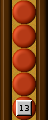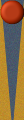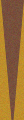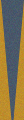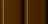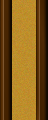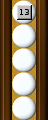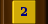How would you go about deciding on cube action here?
You may be surprised that your thoughts regarding the final decision could come down to something as concise as:
9 – 19/2 is negative
(and minus something remains negative)
So I don’t double.

Assumptions

• Money play: the winner wil gain a multiple of the cube value.
• "Pure" 2-roll bear-off: both players are expected to bear off all their men in at most 2 rolls.
Which is not necessarily the case if you randomly drop 2 men in your home board. The (small) correction needed for the (minority of) positions for which this consideration is relevant are kept out of scope here.

Problem classification

Let's call both players A (the one on roll) and B.
Exactly one of the following 13 events will occur:
1. A rolls and wins
2. A rolls and misses; B rolls and wins
3. A rolls and misses; B rolls and misses
4. A rolls and misses; B doubles, A passes
5. A rolls and misses; B doubles, A takes, B rolls and wins
6. A rolls and misses; B doubles, A takes, B rolls and misses
7. A doubles, B passes
8. A doubles, B takes, A rolls and wins
9. A doubles, B takes, A rolls and misses; B rolls and wins
10. A doubles, B takes, A rolls and misses; B rolls and misses
11. A doubles, B takes, A rolls and misses; B redoubles, A passes
12. A doubles, B takes, A rolls and misses; B redoubles, A takes, B rolls and wins
13. A doubles, B takes, A rolls and misses; B redoubles, A takes, B rolls and misses
(4, 5 and 6 are impossible when A owns the cube)
Let RA and RB be the number of rolls (out of 36) that bear off both player's men (A's and B's, respectively) in one throw.
In a spreadsheet, we could calculate the players' optimal cube actions for all values of RA and RB.
But that wouldn't help us behind the board...

The following considerations will help our attempt to classify the situations in a meaningful way:
• If RB < 18, player B will never redouble
• If 18 < RB < 27, player B will redouble when he has the chance, and player A will have to take
• If RB > 27, player B will redouble when he has the chance, and player A will have to drop
• If A owns the cube, he has the option of playing till the end (not allowing B to redouble)
• If B ponders about taking the cube, it makes no difference whether it was an initial cube or a recube by A
And in each situaton, we want criteria for deciding on each of the following questions:
• Is it an initial double for A?
• Is it a redouble for A?
• Is it a drop for B?

Equity function

Suppose you agreed to play this game for €18.
Then your expected gain is somewhere between -18 and +18.
If player B would be certain to bear off if he gets to roll (RB = 36 : imagine both of B's men on the 1 point), A’s winning chance solely depends on his RA (immediately finishing rolls).
Then RA-18 is exactly what player A, on average, expects to win right before his final throw.

This 1/18th of a point is the unity of equity we will use in further calculations (we could refer to it as "roll units" if you want).
And we express the player’s abilities to take 2 men out in 1 throw in this manner:
aRA - 18
bRB - 18

Now what would be the relation between those numbers (a, b) and player A’s equity if the game is played till the end without doubling cube?

One method for determining A’s resulting equity would start from the observation that player B’s winning depends on 1) A missing, followed by 2) B bearing off.
Concluding that A wins with probability P = 1 - (1 - RA/36) RB/36
and his equity (in our “roll units”) would be 18 (2P-1).

Another method determines the weighted sum, for all events, of the resulting expected equities:
A wins (prob. RA/36, result 18)
OR A misses (prob. 1 – RA/36) after wich:
B wins (prob. RB/36, result -18)
OR B misses (prob. 1 – RB/36, result 18)

More formally:After substituting (a+18) and (b+18) for A and B, respectively, and some tedious hocus-pocus, we find: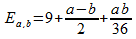We will call this our equity function.

Tip
To get your R number of bearing-off rolls, you could count or:
H = position of the higher man
L = position of the lower man
R = (6 + H - 2L) (7 - H) + 4
Add 1 if 22 bears off too
Add 1 if 11 bears off too

2-Roll bear-off decision table

Let:
END = A's equity if the cube is in the middle and A chooses not to double (ND = "no double"),
ENR = A's equity if A owns the cube and he chooses not to double (NR = "no redouble"),
ED = A's equity if A doubles and B takes.

Now our original cube-handling questions can be answered with:
A offers an initial double if ED > END.
A redoubles if ED > ENR.
B drops if ED > 18

The most boring part is left out here (left to the reader).
The relevant equities, and more importantly, the final decision criteria, are summarized in this table:

 END ENR ED A Doubles if A Redoubles if B Drops if RB < 18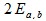always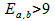18 < RB < 27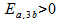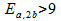RB > 27Note that the expressions Ea,nb are calculated by evaluating our cubeless equity function Ea,b with n-fold b value.
(Especially the redouble question in the case 18 < RB < 27 is answered elegantly with n=3. No need to evaluate two different equities to be compared).

Decision regions

In the following table, the horizontal and vertical axes correspond to RA and RB, respectively.

Legend:
 No double Initial double (but no redouble) Redouble Drop for B

 11,12 36 13 34 14 29 X 22 26 23 25 15,24 23 Z 25 19 33,34 17 Y 16 15 35 14 26 13 44 11 36,45 10 46 8 55,66 6 66 4 4 6 8 10 11 13 14 15 17 19 23 25 26 29 34 36 66 55 66 46 36 45 44 26 35 16 33 34 25 15 24 23 22 14 13 11 12

Position X has been used as example in the so-called "Jacoby paradox".
It is not a redouble for A. But making B's position any better allows A to redouble.

Position Y is an example of the impurity of the model here described.
If A has men on 3 and 4, the possibility of rolling two consecutive 21's makes this a very narrow take for B (as opposed to it being shown in the red region).

Position Z, finally, is the situation with which this article started.
Being the case 18 < B < 27 with the cube on A's side, deciding whether to redouble translates to determining if the equity function, with tripled b value, is positive.
Our parameters (a, b) being (-4, 5), the first two operands in the expression gives us: 9 + (-4-15)/2 = -0.5
The last term is always negative if a and b have different signs - no need to go any further: hold that cube!

Beavers

It is clear that B should beaver if A's equity would be negative after B's simply taking the cube.
These are exactly the situations where A would not have an initial double.
Take care: an unjustified redouble by A does not automatically ask for a beaver: it shouldn't have been an initial cube in the first place.